Go back to  'Complex-Numbers'

In light of the remarks made above, let us give a geometrical significance to the operation of adding complex numbers.

Consider the following two complex numbers:

\begin{align}&{z_1} = {a_1} + i{b_1}\\&{z_2} = {a_2} + i{b_2}\end{align}

These are plotted in the complex plane below: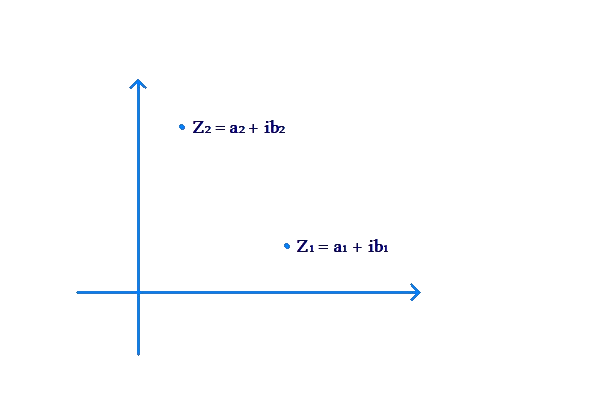How do we geometrically plot the complex number $${z_1} + {z_2}$$? The first step is to think of $${z_1}$$ and $${z_2}$$ and vectors. If you join the origin to $${z_1}$$, you have the vector $${z_1}$$. Similarly, if you join the origin to $${z_2}$$, you have the vector $${z_2}$$: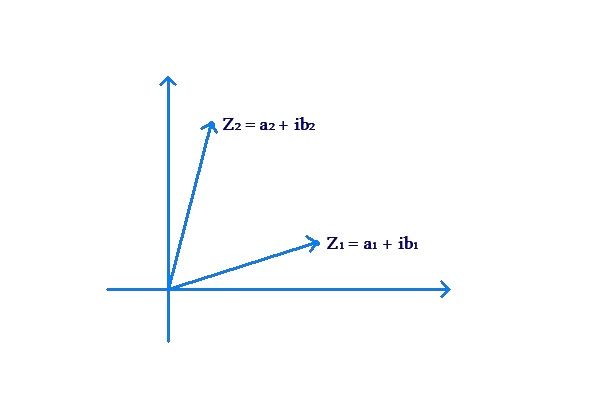Now, these two vectors can be added using the parallelogram law of vector addition: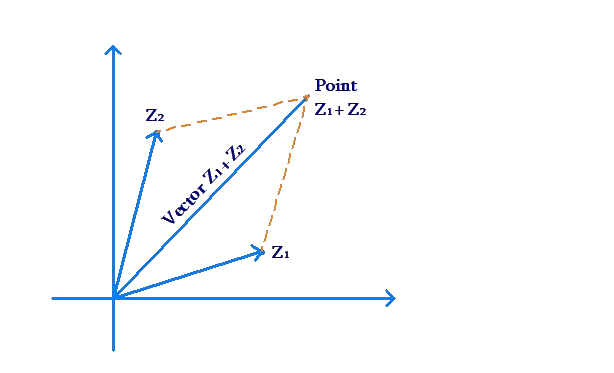The resultant vector represents the vector $${z_1} + {z_2}$$. Its tip represents the point $${z_1} + {z_2}$$.

Let us apply this to a specific example. Take $${z_1} = 2 + 3i$$ and $${z_2} = - 2 + i$$. These two points are plotted below: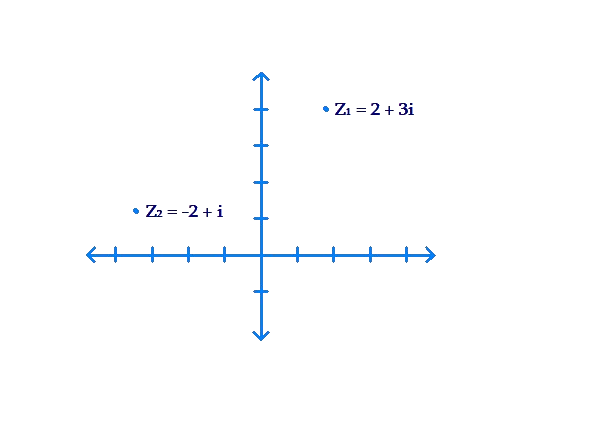To add the two complex numbers, let’s first think of them as vectors: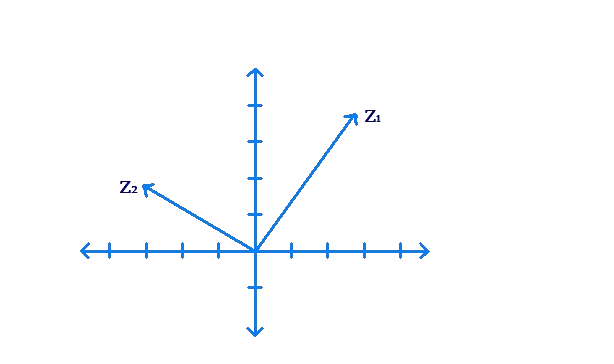Next, we add these two vectors using the parallelogram law. The resultant vector represents the vector ; its tip represents the point $${z_1} + {z_2}$$, or the point $$4i$$: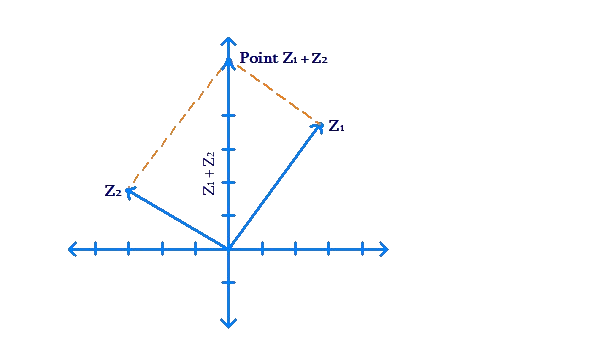The addition result can be verified algebraically:

${z_1} + {z_2} = \left( {2 + 3i} \right) + \left( { - 2 + i} \right) = 4i$

Example 1: Geometrically add the complex numbers $${z_1} = - 1 + 3i$$ and $${z_2} = 4 - i$$.

Solution: Consider the following figure: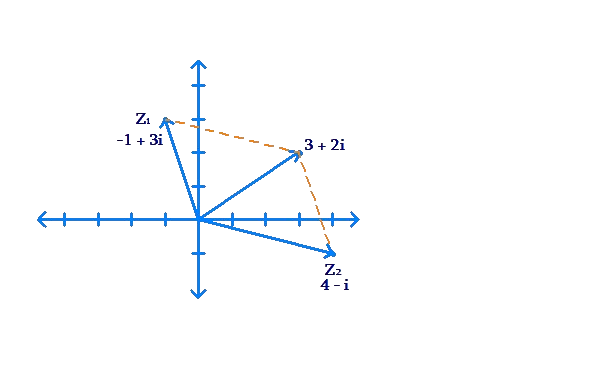Clearly,

${z_1} + {z_2} = 3 + 2i$

You can verify this algebraically.

Complex Numbers
grade 10 | Questions Set 1
Complex Numbers
Complex Numbers
Complex Numbers
grade 10 | Questions Set 2